Published: 30 June 2019

# Vibroimpact mechanism in one separate case

K. Ragulskis1
L. Ragulskis2
1Kaunas University of Technology, K. Donelaičio str. 73, LT-44249, Kaunas, Lithuania
2Vytautas Magnus University, Vileikos str. 8, LT-44404, Kaunas, Lithuania
Corresponding Author:
K. Ragulskis
Views 99

#### Abstract

It is known that in the vibroimpact system at the chosen values of parameters linear relationship between impact velocities and eigenfrequencies may exist. The purpose of this paper is to reveal the qualities of the systems of this type. Investigations are performed by analytical and numerical methods. It is determined that in the systems of this type nonlinear solutions with infinite series of harmonics exist. Multivalued stable and unstable regimes do not exist in the systems. The obtained analytical relationships enabled to reveal new qualities of the systems and to make useful conclusions.

## 1. Introduction

Investigation of dynamics of vibroimpact systems is presented in a number of publications, where the main fundamental achievements are presented including recent years for the cases when the vibrations of the system are with stiff and soft stiffness characteristics. Here the system of intermediate type is investigated. Investigations were performed also graphically, and they enabled to reveal the qualities of the system, which enable to create energetically more effective mechanisms.

Resonances and velocity jumps in nonlinear dynamics are investigated in . Basic theory of vibrating systems with impacts is presented in . Vibro-impact dynamics under periodic and transient excitations is investigated in . Modeling of nonlinear dynamics of a system with clearance is performed in . Dynamical behavior of a vibro-impact oscillator is investigated in . Stabilization of periodic nonlinear systems is analysed in . Basic ideas of vibrating systems in engineering are presented in . Contemporary methods of vibration theory are described in . Basic concepts of mechanical vibrations are presented in . Nonlinear dynamics of inertial actuators is investigated in . Nonlinear effects in dynamics of bearings are presented in . Nonlinear contact dynamics of ultrasonic actuator is investigated in . Non-sinusoidal dynamics of interacting oscillators is analysed in . Free vibration analysis of piezoelectric cylinder is performed in . Free vibrations of nonlinear oscillators are investigated in . Synchronization of impacting mechanical systems is analysed in . Chatter in mechanical systems with impacts is investigated in . Dynamics of systems with impact and friction is analysed in . Periodic orbits of mechanical systems with impacts are investigated in . Vibro-impact nonlinear behavior and energy transfer are described in . Modeling of particle impact is performed in . Impacts in novel mechanisms and their applications are investigated in . Resonant type impact mechanism is analysed in . Positioning using impact drive mechanism is investigated in . Impact mechanics of collisions and experimental results are analysed in . Nonlinear rotor system with vibration absorbers is investigated in . Active vibration absorber for impulse excitation is described in . Nonlinear vibrations of a beam with piezoelectric actuators are investigated in . Nonlinear effects and their use for vibration isolation are analysed in . Nonlinear vibration absorber is investigated in . Nonlinear free vibrations of beams are analysed in . Electro-mechanical coupling vibrations of structures are investigated in . Nonlinear dynamic analysis of vehicle system is performed in . Wideband vibration attenuation is investigated in . Nonlinear vibrations with interactions are analysed in . Piezoelectric nonlinear vibrations are investigated in . Nonlinear analysis of free vibrations of beams is performed in . Nonlocal free and forced vibrations of beams are investigated in . Vibration system with nonlinear coupling is analysed in . Nonlinear vibrations of a system with piecewise linear spring are investigated in . Nonlinear vibrations of piezoelectric plates are analysed in . Free and forced nonlinear vibrations of beams are investigated in . Nonlinearities in piezoceramic actuators are analysed in . Nonlinear free and forced vibrations of beams are investigated in . Nonlinear free vibrations of shells are analysed in . Nonlinear free vibrations of plates are investigated in .

The system is described in the following way:

1
$m\stackrel{¨}{x}+H\stackrel{˙}{x}+Cx=F\mathrm{s}\mathrm{i}\mathrm{n}\omega t,\mathrm{}\mathrm{}\mathrm{}x<0,$
2
${\stackrel{˙}{x}}^{+}=-R{\stackrel{˙}{x}}^{-},\mathrm{}\mathrm{}\mathrm{}x=0,$

where the collision of the vibrating mass is considered as an instantaneous process, ${\stackrel{˙}{x}}^{-}$ denotes velocity before the impact and ${\stackrel{˙}{x}}^{+}$ denotes velocity after the impact, the coefficient of restitution of the impact velocity of the mass is denoted as $R$ and it is in the interval 0 $\le R\le$ 1.

The equation is rearranged:

3
$\stackrel{¨}{x}+2h\stackrel{˙}{x}+{p}^{2}x=f\mathrm{s}\mathrm{i}\mathrm{n}\omega t,\mathrm{}\mathrm{}\mathrm{}x<0,$

where:

$2h=\frac{H}{m},\mathrm{}\mathrm{}\mathrm{}p=\sqrt{\frac{C}{m}},\mathrm{}\mathrm{}\mathrm{}f=\frac{F}{m}.$

## 2. Conservative motion of the system, decaying vibrations when $\mathbit{f}=0$

It is assumed that the impact number $i$ of the mass $m$ to the support takes place when:

4
$t=0,\mathrm{}\mathrm{}\mathrm{}x=0,\mathrm{}\mathrm{}\mathrm{}\stackrel{˙}{x}={\stackrel{˙}{x}}_{i}^{-}>0,$

and the next impact number $i+$ 1 takes place when:

5
$t={T}_{i},\mathrm{}\mathrm{}\mathrm{}x=0,\mathrm{}\mathrm{}\mathrm{}\stackrel{˙}{x}={\stackrel{˙}{x}}_{i+1}^{-}>0.$

According to the Eq. (3) motion after $t\ge$ 0 is:

6
$x={e}^{-ht}\left({C}_{1}\mathrm{c}\mathrm{o}\mathrm{s}\sqrt{{p}^{2}-{h}^{2}}t+{C}_{2}\mathrm{s}\mathrm{i}\mathrm{n}\sqrt{{p}^{2}-{h}^{2}}t\right),$

where the constant quantities ${C}_{1}$ and ${C}_{2}$ are found from the conditions Eq. (4) by assuming ${\stackrel{˙}{x}}_{i}^{+}$ after impact according to the Eq. (4).

Thus:

7
$x=-\frac{R{\stackrel{˙}{x}}_{i}^{-}}{\sqrt{{p}^{2}-{h}^{2}}}{e}^{-ht}\mathrm{s}\mathrm{i}\mathrm{n}\sqrt{{p}^{2}-{h}^{2}}t,$
8
$\stackrel{˙}{x}=-\frac{R{\stackrel{˙}{x}}_{i}^{-}}{\sqrt{{p}^{2}-{h}^{2}}}{e}^{-ht}\left(-h\mathrm{s}\mathrm{i}\mathrm{n}\sqrt{{p}^{2}-{h}^{2}}t+\sqrt{{p}^{2}-{h}^{2}}\mathrm{c}\mathrm{o}\mathrm{s}\sqrt{{p}^{2}-{h}^{2}}t\right),$
9
$\stackrel{¨}{x}=\frac{R{\stackrel{˙}{x}}_{i}^{-}}{\sqrt{{p}^{2}-{h}^{2}}}{e}^{-ht}\left[\left({p}^{2}-{h}^{2}\right)\mathrm{s}\mathrm{i}\mathrm{n}\sqrt{{p}^{2}-{h}^{2}}t+2h\sqrt{{p}^{2}-{h}^{2}}\mathrm{c}\mathrm{o}\mathrm{s}\sqrt{{p}^{2}-{h}^{2}}t\right].$

The next impact number $i+$ 1 takes place at the conditions Eq. (5). By taking into account the Eqs. (7) and (8) it is obtained:

10
${T}_{i}=\frac{\pi }{\sqrt{{p}^{2}-{h}^{2}}}.$

During the time of the cycle of motion between the impacts $i$ and $i+$ 1 the change of velocities is lost, which is estimated by the dummy coefficient:

11
${R}_{{f}_{i+1,i}}=\frac{{\stackrel{˙}{x}}_{i+1}^{-}}{{\stackrel{˙}{x}}_{i}^{-}}=+R\cdot \mathrm{exp}\left(-\frac{h}{\sqrt{{p}^{2}-{h}^{2}}}\pi \right)=-R\cdot \mathrm{exp}\left(\frac{h/p}{\sqrt{1-{\left(h/p\right)}^{2}}}\right).$

Further graphical material representing dynamics of the investigated system is presented for various parameters of the system in Fig. 1, Fig. 2 and Fig. 3.

Fig. 1Dynamics of the system when the initial conditions of motion t= 0, x0= 0, x˙0= –1 and p= 1 for h= 0 (thin line), h= 0.25 (line of medium thickness) and h= 0.5 (thick line)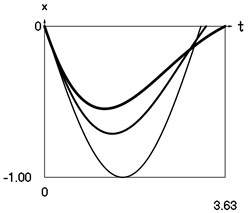a) Displacement as function of time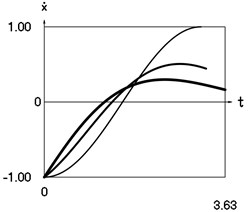b) Velocity as function of time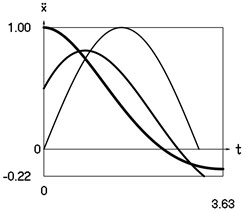c) Acceleration as function of time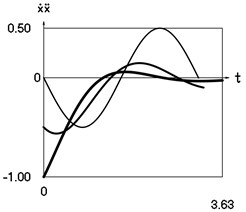d) Velocity multiplied by acceleration as function of time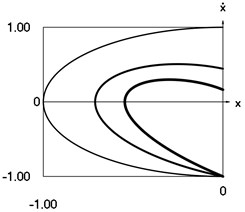e) Phase trajectory: velocity as function of displacement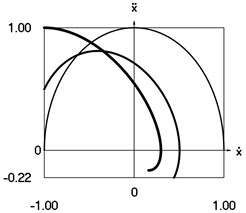f) Phase trajectory: acceleration as function of velocity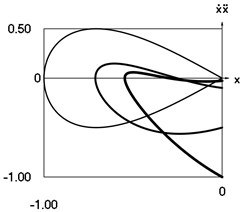g) Phase trajectory: velocity multiplied by acceleration as function of displacement

Fig. 2Dynamics of the system when h= 0 and p= 1 for the initial conditions of motion t= 0, x0= 0, x˙0= –1 (thin line), t= 0, x0= 0, x˙(0)= –2/3 (line of medium thickness) and t= 0, x0= 0, x˙(0)= –1/3 (thick line)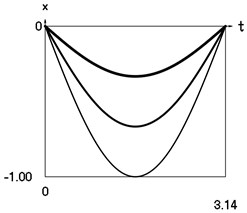a) Displacement as function of time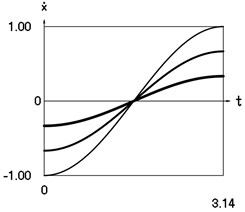b) Velocity as function of time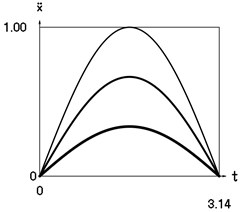c) Acceleration as function of time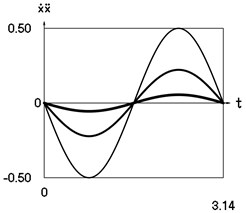d) Velocity multiplied by acceleration as function of time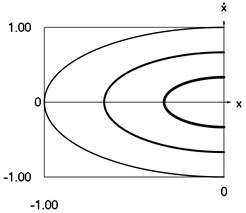e) Phase trajectory: velocity as function of displacement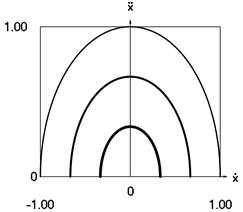f) Phase trajectory: acceleration as function of velocity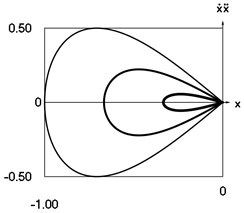g) Phase trajectory: velocity multiplied by acceleration as function of displacement

## 3. Dynamics of the conservative system

Case: conservative system, that is when:

12
$h=f=0,\mathrm{}\mathrm{}\mathrm{}R=1.$

In this case the Eqs. (7)-(10) take the following form when:

13
$x<0,$
14
$x=-\frac{{\stackrel{˙}{x}}^{-}}{p}\mathrm{s}\mathrm{i}\mathrm{n}pt,$
15
$\stackrel{˙}{x}=-{\stackrel{˙}{x}}^{-}\mathrm{c}\mathrm{o}\mathrm{s}pt,$
16
$\stackrel{¨}{x}={\stackrel{˙}{x}}^{-}p\mathrm{s}\mathrm{i}\mathrm{n}pt,$
17
$\stackrel{-}{T}=\frac{\pi }{p}.$

Period of motion $\stackrel{-}{T}$ is the eigenperiod of vibrations of the system and $\stackrel{-}{\omega }$ is the eigenfrequency of vibrations of the system, that is by equating the right sides of the Eqs. (17) it is obtained:

18
$\stackrel{-}{\omega }=2p,\mathrm{}\mathrm{}\mathrm{}\stackrel{-}{T}=\frac{\pi }{p}=\frac{2\pi }{\stackrel{-}{\omega }}.$

Fig. 3Dynamics of the system when h= 0.5 and p= 1 for the initial conditions of motion t= 0, x0= 0, x˙0= –1 (thin line), t= 0, x0= 0, x˙(0)= –2/3 (line of medium thickness) and t= 0, x0= 0, x˙0= –1/3 (thick line)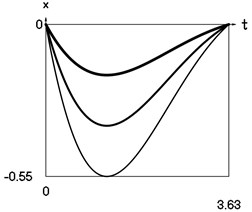a) Displacement as function of time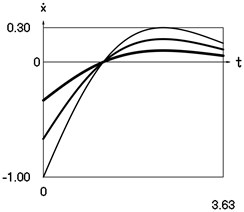b) Velocity as function of time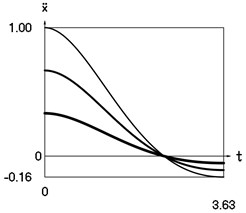c) Acceleration as function of time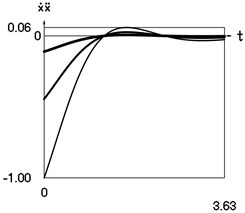d) Velocity multiplied by acceleration as function of time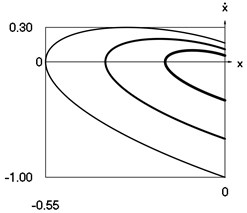e) Phase trajectory: velocity as function of displacement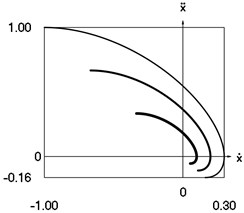f) Phase trajectory: acceleration as function of velocity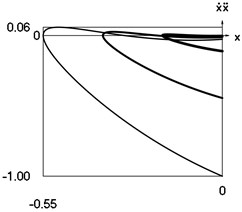g) Phase trajectory: velocity multiplied by acceleration as function of displacement

By expanding the functions of displacement, velocity and acceleration into the Fourier series the following expressions of the first terms of the series are obtained. The laws of motions may be expanded into the Fourier series. From the Eqs. (14-16) it is obtained:

19
$x=-\frac{{\stackrel{˙}{x}}^{-}}{p}\left\{\frac{2}{\pi }-\frac{4}{\pi }{\sum }_{n=1}^{n=\mathrm{\infty }}\left[\frac{1}{\left(2n-1\right)\left(2n+1\right)}\mathrm{c}\mathrm{o}\mathrm{s}n\stackrel{-}{\omega }t\right]\right\},$
20
$\stackrel{˙}{x}=-{\stackrel{˙}{x}}^{-}\frac{8}{\pi }{\sum }_{n=1}^{n=\mathrm{\infty }}\left[\frac{n}{\left(2n-1\right)\left(2n+1\right)}\mathrm{s}\mathrm{i}\mathrm{n}n\stackrel{-}{\omega }t\right],$
21
$\stackrel{¨}{x}=p{\stackrel{˙}{x}}^{-}\left\{\frac{2}{\pi }-\frac{4}{\pi }{\sum }_{n=1}^{n=\mathrm{\infty }}\left[\frac{1}{\left(2n-1\right)\left(2n+1\right)}\mathrm{c}\mathrm{o}\mathrm{s}n\stackrel{-}{\omega }t\right]\right\},$

where $\stackrel{-}{\omega }=2p.$

From the Eqs. (14) and (15) it is obtained:

22
${\stackrel{˙}{x}}^{2}+{\left(px\right)}^{2}={\left({\stackrel{˙}{x}}^{-}\right)}^{2},$

that is in the system of coordinates $\stackrel{˙}{x}0px$ there is a circle the length of radius of which is equal to ${\stackrel{˙}{x}}^{-}.$

From the Eqs. (15) and (16) it is obtained:

23
${\left(\frac{\stackrel{¨}{x}}{p}\right)}^{2}+{\stackrel{˙}{x}}^{2}={\left({\stackrel{˙}{x}}^{-}\right)}^{2},$

that is in the system of coordinates $\frac{\stackrel{¨}{x}}{p}0\stackrel{˙}{x}$ there is a circle the length of radius of which is equal to ${\stackrel{˙}{x}}^{-}.$

Further graphical material of amplitude frequency characteristics is presented in Fig. 4.

Fig. 4Amplitude frequency characteristics (constant part and first three harmonics) when h= 0 and p= 1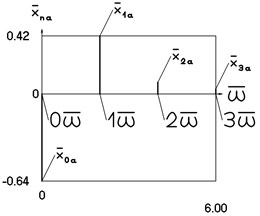a) Displacement frequency $\mathrm{c}\mathrm{o}\mathrm{s}$ characteristic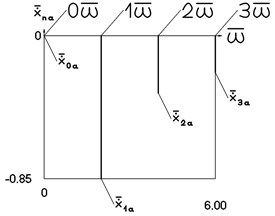b) Velocity frequency $\mathrm{s}\mathrm{i}\mathrm{n}$ characteristic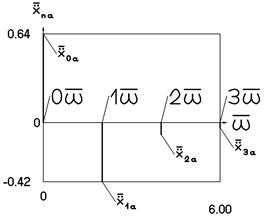c) Acceleration frequency $\mathrm{c}\mathrm{o}\mathrm{s}$ characteristic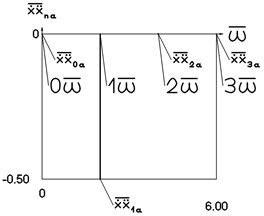d) Velocity multiplied by acceleration frequency $\mathrm{s}\mathrm{i}\mathrm{n}$ characteristic

## 4. Conclusions

On the basis of the presented results the qualities of dynamic behavior of the nonlinear vibroimpact mechanism in separate case, when the contacting surface of the vibrating part of the system with the impacting surface is in the position of static equilibrium, are investigated.

Analytical relationships describing the motion of the system and amplitude frequency characteristics have been determined and are presented in the paper. Graphical relationships for typical parameters of the system were obtained and are investigated. It is shown that the values of eigenfrequencies of vibroimpact vibrations do not depend on the values of amplitudes of excitations. Because of this fact multivalued stable and unstable regimes can not take place in the vicinities of resonances.

The presented results enable to perform the design of vibrating vibroimpact systems of this type.